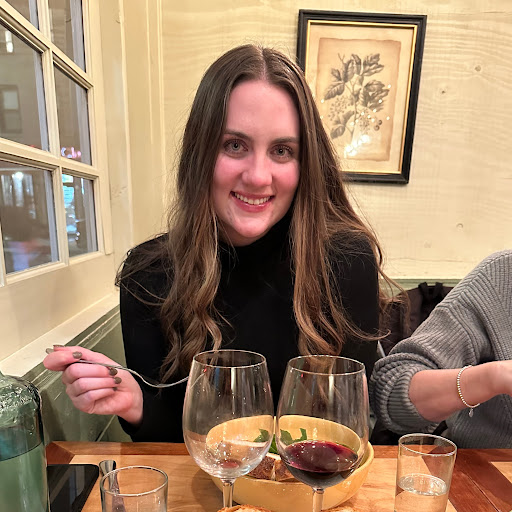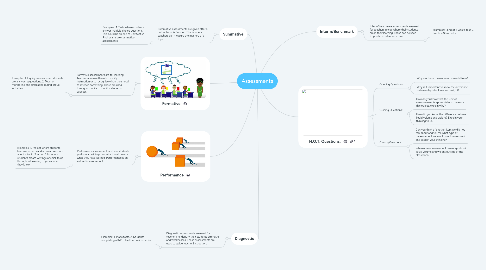# AssessmentsCaitlin Ferris
Get Started. It's FreeAssessments## 1. Formative

### 1.1. Formative assessments are for learning. Teachers can use these to modify instruction or to recognize when they need to reteach something. These are used throughout units to monitor students success.

1.1.1. Examples: 1. Inquiry lessons when students are answering questions. 2. Teacher monitoring and circulating during group activities.

## 2. Summative

### 2.1. Summative assessments are given after a unit or topic is finished. This is where teachers can measure the mastery of a topic.

2.1.1. Examples: 1. Tests where students answer multiple choice questions. This could be an end of unit test. 2. Final exams are summative assessments.

## 3. Interm/Benchmark

### 3.1. Interm/Benchmark assessments are used for teachers to see where their students are in their learning. These can be used to predict student outcome.

3.1.1. Examples: 1.End of 6 weeks test. 2. Practice Starr tests.

## 4. Performance

### 4.1. Performance assessment is where students preform a task to prove what they know or what they have learned. Performance is an authentic assessment.

4.1.1. Examples: 1. Project where students become experts and answer questions about a topic. 2. A portfolio where students create writings or small tasks throughout learning to prove what they know.

## 5. Diagnostic

### 5.1. Diagnostic assessments are used for teachers to identify their students strengths and weaknesses. These assessments are used to guide teacher instruction.

5.1.1. Examples: 1. Maps tests. 2. Students completing a KWL chart before instruction.

## 6. H.O.T. Questions:

### 6.1. Opening Questions:

6.1.1. Why are these measurements useful to us?

6.1.2. Why is it important to know the difference between liquid volume and weight?

### 6.2. Guiding Questions:

6.2.1. How do you know that this unit of measurement is appropriate to measure this object, explain why?

6.2.2. How did you know the difference between liquid volume and weight? Explain your thinking to us.

### 6.3. Closing Questions:

6.3.1. Can you think of another item we did not talk about and tell me what type of measurement we would use to measure it and explain your thinking?

6.3.2. Where can you use your knowledge about liquid volume and weight outside of the classroom?